# Introduction to SPSS

This page looks at how to conduct some common inferential statistical tests in SPSS (for more information on any of these tests, you may also like to visit the Introduction to statistics module). In particular, it covers the following (use the drop-down menu above to jump to a different section as required):

• Obtaining and interpreting confidence intervals
• Testing for differences in means with $$t$$ tests and one-way ANOVA
• Testing for association with the Chi-square test
• Testing for linear correlation with Pearson’s correlation coefficient

The examples covered make use of the Household energy consumption data.sav file, which contains fictitious data for 80 people based on a short ‘Household energy consumption’ questionnaire. If you want to work through the examples provided you can download the data file using the following link:

If you would like to read the sample questionnaire for which the data relates, you can do so using this link:

Before commencing the analysis, note that the default is for dialog boxes in SPSS to display any variable labels, rather than variable names. You may find this helpful, but if you would prefer to view the variable names instead then from the menu choose:

• Edit
• Options…
• Change the Variable Lists option to Display names

## Determining a confidence interval

A confidence interval is a range of probable values for an unknown population parameter, based on the sample statistic (for example the mean). The percentage associated with the confidence interval (usually $$95\%$$) is termed the confidence coefficient , and this is the level of confidence you have that the range actually includes the true value. This section details how to use SPSS to calculate and interpret a $$95\%$$ confidence interval for the mean of a continuous variable, but if you would like more information on confidence intervals you may first like to visit the Introduction to statistics module.

A question you may wish to ask of the data is: Based on the data observed in the sample, what is the $$95\%$$ confidence interval for the population mean summer energy consumption?

Before calculating this confidence interval in SPSS, it is important to note that some assumptions need to be met when using a confidence interval to estimate the mean of a population. These assumptions are as follows:

Assumption 1: The sample is a random sample that is representative of the population.

Assumption 2: The observations are independent, meaning that measurements for one subject have no bearing on any other subject’s measurements.

Assumption 3: The variable is normally distributed, or the sample size is large enough to ensure normality of the sampling distribution.

While these first two assumptions should be met during the design and data collection phases, the third assumption should be checked at this stage. For instructions on doing this in SPSS, see the normal distribution page of this module.

If all assumptions are met (as is the case for this example), you can obtain a $$95\%$$ confidence interval for the mean in SPSS by choosing the following from the menu (either from the Data Editor or Output window; note that you may already have this output as part of testing for normality):

• Analyze
• Descriptive statistics
• Explore…
• move the variable (for example the ‘q6’ variable) into the Dependent List (remove other variables if required)
• select Statistics in the Display section underneath
• click on OK

The output should then include the following: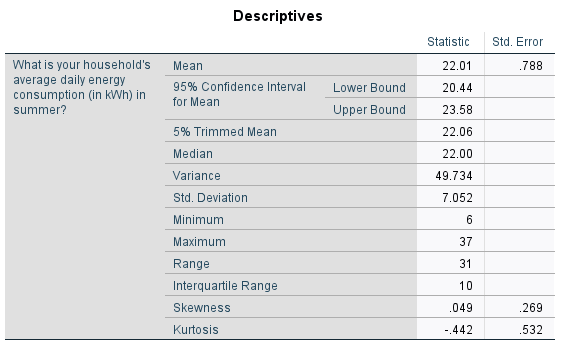This tells us that based on what has been observed in the sample, we can be $$95\%$$ confident that the mean summer energy consumption of the wider population is somewhere between $$20.44$$ kWh (lower bound) and $$23.58$$ kWh (upper bound).

Note that to calculate a confidence interval with a confidence coefficient other than $$95\%$$, follow the same instructions but also click on the Statistics button and change the Confidence Interval for Mean as required.

## Hypothesis testing

Hypothesis testing involves testing statements (hypotheses) about the population using data collected in the sample. The particular test to use depends on the nature of the hypothesis, and there are often versions of each test that are parametric (assume normal distribution and require at least one continuous variable) and non-parametric (don’t assume normal distribution and can be used for ordinal variables). If you would like more information on hypothesis testing, you may like to visit the Introduction to statistics module.

The remainder of this page details how to use SPSS to perform some of the most frequently used tests, which are applicable in the following situations (for more information on some of the tests, click on the link provided):

Situation Parametric test Non-parametric test
You wish to test whether a sample comes from a population with a specific mean One sample $$t$$ test One sample Wilcoxon signed-rank test (compares the median)
You wish to compare the means of two related groups Paired samples $$t$$ test Wilcoxon signed rank test (compares mean ranks)
You wish to compare the means of two unrelated groups Independent samples $$t$$ test Mann-Whitney U test (compares mean ranks)
You wish to compare the means of more than two unrelated groups One-way ANOVA Kruskal-Wallis one-way ANOVA (compares mean ranks)
You wish to test whether there is association between two variables   Chi-square test
You wish to test whether there is a linear correlation between two variables Pearson’s correlation Spearman’s Rho or Kendall’s Tau-B (can test for non-linear correlation)

Whichever test you are using, it is important to note that conducting the test in SPSS is just part of the process. In particular, the recommended steps to follow in order to successfully conduct a hypothesis test are listed below.

1. Determine the hypothesis to be tested
2. Establish the appropriate test for the hypothesis
3. Check that all assumptions for the test are valid (SPSS can help with some of these)
4. Use SPSS to conduct the relevant test and determine the $$p$$ value and confidence interval (if applicable)
5. Interpret the result

Examples of how to do this for each of the tests in the table are covered in the sections that follow.

## Conducting a one sample $$t$$ test

A question you may wish to ask of the wider population is: Does this sample come from a population where the mean summer daily energy consumption is $$19$$kWh?

This question can be answered by following the recommended steps, as follows:

1. The appropriate hypotheses for this question are:
$$\textrm{H}_\textrm{0}$$: The sample comes from a population with a mean summer daily energy consumption of $$19$$kWh
$$\textrm{H}_\textrm{A}$$: The sample does not come from a population with a mean summer daily energy consumption of $$19$$kWh

2. The appropriate test to use is the one sample $$t$$ test, as we are testing whether the sample comes from a population with a specific mean ($$19$$kWh in this case).

3. The assumptions for a one sample $$t$$ test are as follows:

Assumption 1: The sample is a random sample that is representative of the population.

Assumption 2: The observations are independent, meaning that measurements for one subject have no bearing on any other subject’s measurements.

Assumption 3: The variable is continuous.

Assumption 4: The variable is normally distributed, or the sample size is large enough to ensure normality of the sampling distribution.

While the first three assumptions should be met during the design and data collection phases, the fourth assumption should be checked at this stage. For instructions on doing this in SPSS, see the normal distribution page of this module.

If the normality assumption is not met you can try transforming the data or conducting the One sample Wilcoxon signed-rank test instead. You can also use this test if you have an ordinal rather than continuous variable.

1. If all the assumptions are met, you can conduct the one sample $$t$$ test in SPSS by choosing the following from the SPSS menu (either from the Data Editor or Output window):
• Analyze
• Compare Means
• One-Sample T Test
• move the variable (‘q6’) into the Test Variable(s) box
• enter the Test Value of 19
• click on OK

The output should look like this: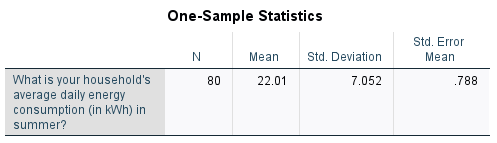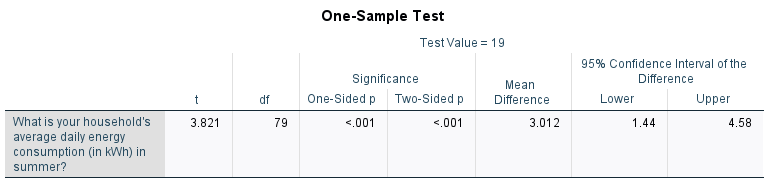1. From the first table we can see that the mean summer daily energy consumption in our sample is $$22.01$$kWh, which is $$3.012$$kWh more than our hypothesised value. To test whether this is a statistically significant difference, we need to refer to the second table and to the $$p$$ value and confidence interval.

While there are actually two $$p$$ values listed in the second table (the ‘One-sided $$p$$’ and ‘Two-sided $$p$$’), the standard one to use is the ‘Two-Sided $$p$$’ value as this is used to test for a difference in either direction (that is, to test whether the mean is significantly greater than or less than $$19$$, as per our alternative hypothesis). Since $$p < .05$$ (in fact $$p < .001$$) and since the $$95\%$$ confidence interval for the difference between the population mean summer daily energy consumption and the hypothesised value does not include zero ($$95\% \textrm{CI}$$ [$$1.44$$kWh, $$4.58$$kWh]), we can reject the null hypothesis and conclude that the sample actually comes from a population with mean summer daily energy consumption significantly more than $$19$$kWh.

Finally, the third table provides the effect sizes, which can be used to test for practical significance. The ‘Point Estimate’ for ‘Cohen’s $$d$$’ of $$0.427$$ indicates a medium effect.

For more information on how to interpret these results see the Introduction to statistics module.

## Conducting a paired samples $$t$$ test

A question you may wish to ask of the wider population is: Is there a statistically significant difference between mean summer daily energy consumption and mean winter daily energy consumption?

This question can be answered by following the recommended steps, as follows:

1. The appropriate hypotheses for this question are:
$$\textrm{H}_\textrm{0}$$: There is no significant difference between mean summer and winter daily energy consumption
$$\textrm{H}_\textrm{A}$$: There is a significant difference between mean summer and winter daily energy consumption

2. The appropriate test to use is the paired samples $$t$$ test, as we are comparing the means of two related groups (summer and winter consumption for the sample people).

3. The assumptions for a paired samples $$t$$ test are as follows:

Assumption 1: The sample is a random sample that is representative of the population.

Assumption 2: The observations are independent, meaning that measurements for one subject have no bearing on any other subject’s measurements.

Assumption 3: The variables are both continuous.

Assumption 4: Both variables as well as the difference variable (the differences between each data pair) are normally distributed, or the sample size is large enough to ensure normality of the sampling distributions.

While the first three assumptions should be met during the design and data collection phases, the fourth assumption should be checked at this stage. For instructions on doing this in SPSS, see the normal distribution and Transformations pages of this module.

If the normality assumption is not met you can try transforming the data or conducting the Wilcoxon signed rank test instead. You can also use this test if you have ordinal rather than continuous variables.

1. If all the assumptions are met, you can conduct the paired samples $$t$$ test in SPSS by choosing the following from the SPSS menu (either from the Data Editor or Output window):
• Analyze
• Compare Means
• Paired-Samples T Test
• move the variables (‘q6’ and ‘q7’) into the Paired Variables box as Pair 1
• click on OK

The output should look like this: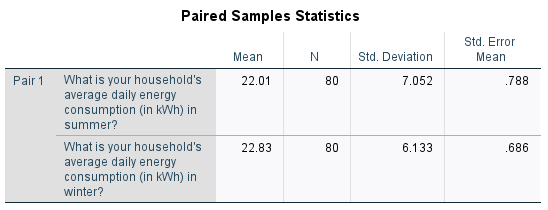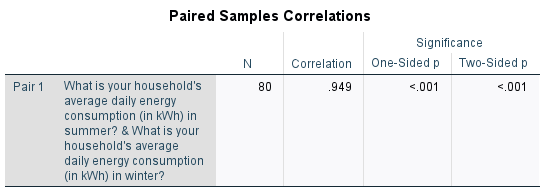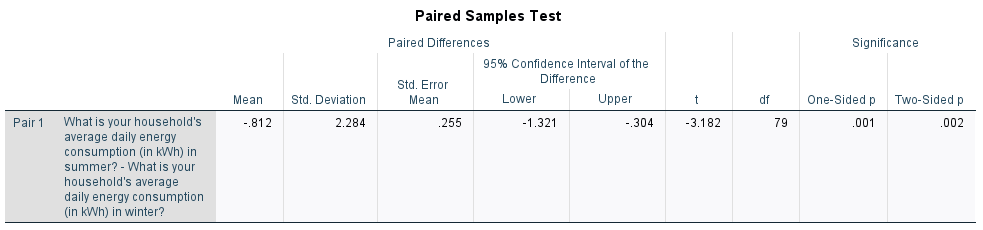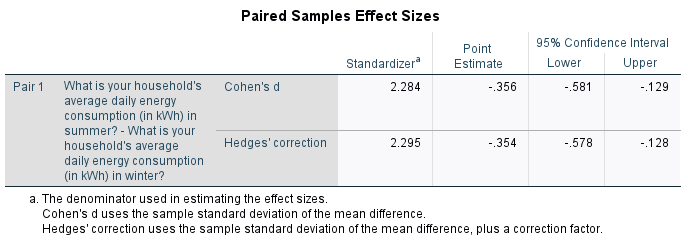1. From the first table we can see that the mean summer daily energy consumption in our sample is $$22.01$$kWh, while the mean winter daily energy consumption is $$22.83$$kWh; a difference of $$0.812$$kWh. To test whether this is a statistically significant difference, we need to refer to the third table and to the $$p$$ value and confidence interval. (Note that the second table provides information about the correlation between the variables, and does not need to be interpreted here.)

While there are actually two $$p$$ values listed in the third table (the ‘One-sided $$p$$’ and ‘Two-sided $$p$$’), the standard one to use is the ‘Two-Sided $$p$$’ value as this is used to test for a difference in either direction (that is, to test whether one mean is significantly greater than or less than the other, as per our alternative hypothesis). Since $$p < .05$$ (in fact $$p= .002$$) and since the $$95\%$$ confidence interval for the difference between the population mean summer and winter daily energy consumptions does not include zero ($$95\% \textrm{CI}$$ [$$-1.321$$kWh, $$-0.304$$kWh]), we can reject the null hypothesis and conclude that the mean summer daily energy consumption is significantly less than the mean winter daily energy consumption.

Finally, the third table provides the effect sizes, which can be used to test for practical significance. The ‘Point Estimate’ for ‘Cohen’s $$d$$’ of $$-0.356$$ indicates a small to medium effect.

For more information on how to interpret these results see the Introduction to statistics module.

## Conducting an independent samples $$t$$ test

A question you may wish to ask of the wider population is: Is there a statistically significant difference in mean summer daily energy consumption for those with and without children?

This question can be answered by following the recommended steps, as follows:

1. The appropriate hypotheses for this question are:
$$\textrm{H}_\textrm{0}$$: There is no significant difference in mean summer daily energy consumption for those with and without children
$$\textrm{H}_\textrm{A}$$: There is a significant difference in mean summer daily energy consumption for those with and without children

2. The appropriate test to use an independent samples $$t$$ test, as we are comparing the means of two unrelated groups (summer consumption of those with and without children).

3. The assumptions for an independent samples $$t$$ test are as follows:

Assumption 1: The sample is a random sample that is representative of the population.

Assumption 2: The observations are independent, meaning that measurements for one subject have no bearing on any other subject’s measurements.

Assumption 3: The dependent variable is continuous.

Assumption 4: The variable is normally distributed for both groups, or the sample size is large enough to ensure normality of the sampling distribution.

While the first three assumptions should be met during the design and data collection phases, the fourth assumption should be checked at this stage. For instructions on doing this in SPSS, see the normal distribution page of this module.

If the normality assumption is not met you can try transforming the data or conducting the Mann-Whitney U test instead. You can also use this test if you have an ordinal rather than continuous dependent variable.

1. If all the assumptions are met, you can conduct the independent samples $$t$$ test in SPSS by choosing the following from the SPSS menu (either from the Data Editor or Output window):
• Analyze
• Compare Means
• Independent-Samples T Test
• move the continuous variable (‘q6’) into the Test Variable(s) box
• move the categorical variable (‘q3’) into the Grouping Variable box
• click on Define Groups…
• keep Group 1 as category 1 and Group 2 as category 2 and select Continue
• click on OK

The output should look like this: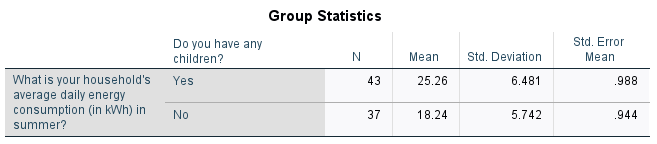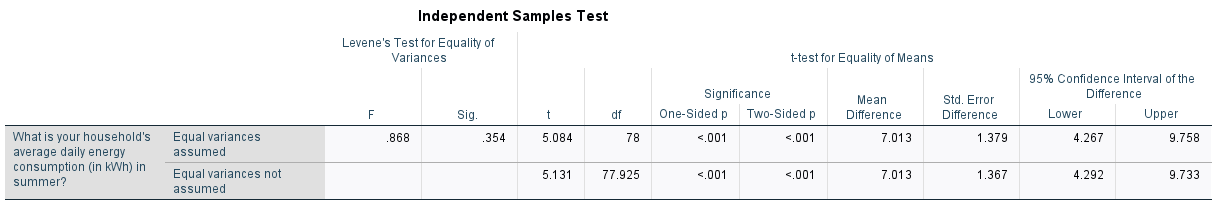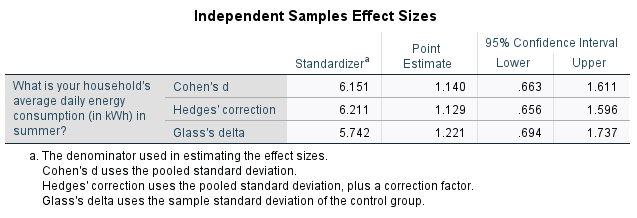1. From the first table we can see that the mean summer daily energy consumption in our sample for those with children is $$25.26$$kWh, while for those without children it is $$18.24$$kWh; a difference of $$7.013$$kWh. To test whether this is a statistically significant difference, we need to refer to the second table and to the $$p$$ value and confidence interval.

The second table actually contains five $$p$$ values, of which we need to assess two. The first is for Levene’s Test for Equality of Variances (listed as ‘Sig.’), and since this $$p > .05$$ (in fact $$p = .354$$), we can assume equal variances. This means we should interpret the top row of the remainder of the table. While there are actually two $$p$$ values listed in the remainder of the top row (the ‘One-sided $$p$$’ and ‘Two-sided $$p$$’), the standard one to use is the ‘Two-Sided $$p$$’ value as this is used to test for a difference in either direction (that is, to test whether one mean is significantly greater than or less than the other, as per our alternative hypothesis). Since $$p < .05$$ (in fact $$p < .001$$) and since the $$95\%$$ confidence interval for the difference between the population mean summer daily energy consumptions of those with and without children does not include zero ($$95\% \textrm{CI}$$ [$$4.267$$kWh, $$9.758$$kWh]), we can reject the null hypothesis and conclude that the mean summer daily energy consumption is significantly more for those with children compared to those without.

Finally, the third table provides the effect sizes, which can be used to test for practical significance. The ‘Point Estimate’ for ‘Cohen’s $$d$$’ of $$1.14$$ indicates a large effect.

For more information on how to interpret these results see the Introduction to statistics module.

## Conducting a one-way ANOVA

A question you may wish to ask of the wider population is: Is there a statistically significant difference in mean summer daily energy consumption for any of the different marital statuses?

This question can be answered by following the recommended steps, as follows:

1. The appropriate hypotheses for this question are:
$$\textrm{H}_\textrm{0}$$: There is no significant difference in mean summer daily energy consumption for any of the different marital statuses
$$\textrm{H}_\textrm{A}$$: The mean summer daily energy consumption of at least one of the marital status groups is significantly different from the others

2. The appropriate test to use a one-way ANOVA, as we are comparing the means of three unrelated groups (summer consumption of those with a marital status of single, married and other).

3. The assumptions for a one-way ANOVA are as follows:

Assumption 1: The sample is a random sample that is representative of the population.

Assumption 2: The observations are independent, meaning that measurements for one subject have no bearing on any other subject’s measurements.

Assumption 3: The dependent variable is continuous.

Assumption 4: The variable is normally distributed for each of the groups, or the sample size is large enough to ensure normality of the sampling distribution.

Assumption 5: The populations being compared have equal variances.

While the first three assumptions should be met during the design and data collection phases, the fourth and fifth assumptions should be checked at this stage. For instructions on checking the normality assumption in SPSS, see the normal distribution page of this module. Instructions on checking the equal variances assumption are included in the analysis stage.

If the normality assumption is not met you can try transforming the data or conducting the Kruskall-Wallis one-way ANOVA instead. You can also use this test if you have an ordinal rather than continuous dependent variable. If the equal variances assumption is violated you will need to use a Welch or Brown-Forsythe statistic instead.

1. If the first four assumptions are met, you can conduct the one-way ANOVA in SPSS by choosing the following from the SPSS menu (either from the Data Editor or Output window):
• Analyze
• Compare Means
• One-Way ANOVA
• move the continuous variable (‘q6’) into the Dependent List box
• move the categorical variable (‘q4’) into the Factor box
• select Options… from the right hand menu
• select Descriptive and Homogeneity of variance test in the dialogue box
• click on Continue
• click on OK

The output should look like this: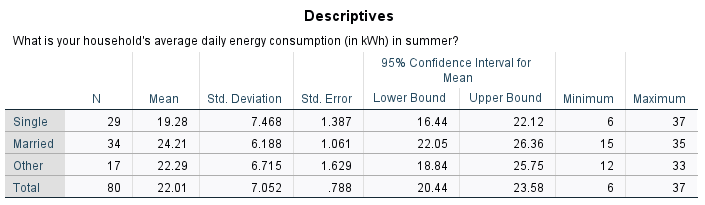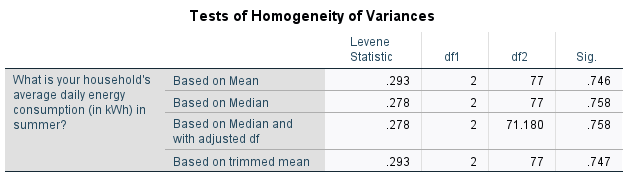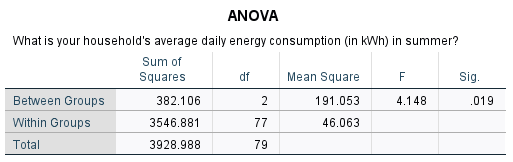1. From the first table we can see that the mean summer daily energy consumption in our sample for those who are single is $$19.28$$kWh, for those who are married it is $$24.21$$kWh and for those who classified their marital status as ‘Other’ it is $$22.29$$kWh. To test whether there are any statistically significant differences between these values, we need to refer to the third table and to the $$p$$ value.

Before this though, we need to use the second table to evaluate the fifth assumption using Levene’s test of homogeneity of variance. The $$p$$ value to evaluate is the one in the ‘Based on Mean’ row, which is listed as ‘Sig.’. Since this $$p > .05$$ (in fact $$p = .746$$), we can assume equal variances and therefore the fifth assumption for the test is met. If the fifth assumption is not met, you can go back through the menu and keep the previous selections, but this time also select either the Brown-Forsythe test or the Welch test in the Options dialogue box.

The third table contains the $$p$$ value to evaluate for the one-way ANOVA, and since $$p < .05$$ (in fact $$p = .019$$) we can reject the null hypothesis and conclude that the mean summer daily energy consumption is significantly different for at least one of the marital status groups.

To find out where the significant difference(s) lie you can conduct a post hoc test. While there are many different options to choose from, a common test to try is Tukey’s HSD test (alternatively, if the homogeneity of variance assumption is violated you can use the Games Howell test). To do this, go back through and keep the previous selections but also do the following:

• select Post Hoc… from the right hand menu
• select Tukey in the dialogue box
• click on Continue
• click on OK

The output should be the same as previously, but with the addition of the following two tables: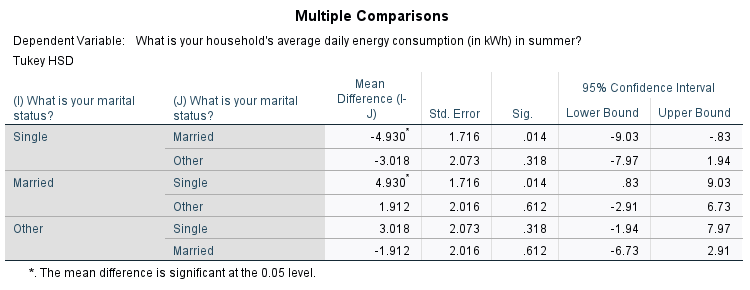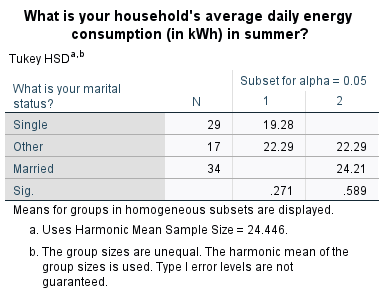Both tables indicate that the only significant difference in mean summer daily energy consumption is between the single and married groups. This is shown by the fact that the $$p < .05$$ (in fact $$p = .014$$) for this pair in the first table, and by the fact that mean values for the single and married groups do not appear in the same column of the second table.

## Testing for association

A question you may wish to ask of the wider population is: Is there a statistically significant association between having children and owning a dishwasher?

This question can be answered by following the recommended steps, as follows:

1. The appropriate hypotheses for this question are:
$$\textrm{H}_\textrm{0}$$: There is no significant association between having children and owning a dishwasher
$$\textrm{H}_\textrm{A}$$: There is significant association between having children and owning a dishwasher

2. The appropriate test to use is a Chi-square test, as we are testing for association between two categorical variables (having children and owning a dishwasher).

3. The assumptions for the Chi-square test are as follows:

Assumption 1: The sample is a random sample that is representative of the population.

Assumption 2: The observations are independent, meaning that measurements for one subject have no bearing on any other subject’s measurements.

Assumption 3: The categories used for the variables are mutually exclusive.

Assumption 4: The categories used for the variables are exhaustive.

Assumption 5: No more than $$20\%$$ of the expected frequencies are less than $$5$$ (if this is violated Fisher’s exact test can be used instead).

While the first four assumptions should be met during the design and data collection phases, the fifth assumption can be checked during the analysis stage. If this assumption is violated and your variables each have only two categories, you can use the results displayed in SPSS for Fisher’s exact test instead. If your variables have more categories, you may be able to exclude or combine some of them. For instructions on combining categories by recoding, see the Transformations page of this module.

1. If the first four assumptions are met, you can conduct the Chi-square test in SPSS by choosing the following from the SPSS menu (either from the Data Editor or Output window):
• Analyze
• Descriptive statistics
• Crosstabs…
• transfer the independent variable (‘q3’) to the Row(s) box
• transfer the dependent variable (‘q16.3’) to the Column(s) box
• select Statistics… from the right hand menu
• select Chi-square and Phi and Cramer’s V in the dialogue box
• click on Continue
• select Cells… from the right hand menu
• select Observed and Expected in the dialogue box
• click on Continue
• click on OK

The output should look like this: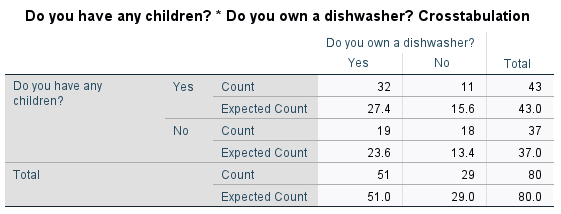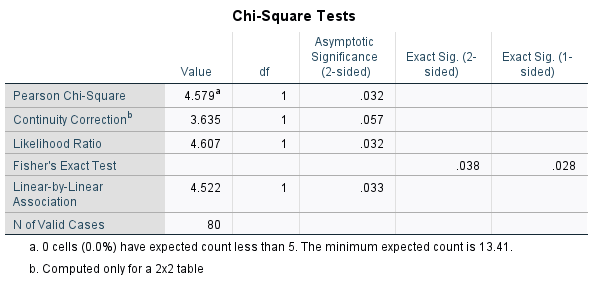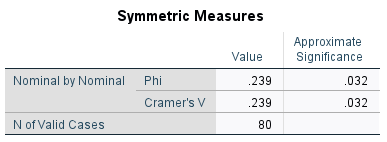1. The first table simply shows that $$80$$ cases have been processed. The second table shows how the actual sample data compares with what would be expected if there was no association between having children and dishwasher ownership. The fact that there is a bit of a difference between the observed and expected values provides evidence of association in the sample, with the nature of the association being that people with children are more likely to own a dishwasher.

To find out whether the association is significant, we need to refer to the third table and to the ‘Asymptotic Significance (2-sided)’ value in the ‘Pearson Chi-Square’ row. Since $$p < .05$$ ($$p = .032$$) we can reject the null hypothesis and conclude that there is a statistically significant association between having children and owning a dishwasher.

Finally, the fourth table provides the effect sizes, which can be used to test for practical significance. The ‘Cramer’s $$V$$’ of $$.239$$ indicates a small to medium effect.

For more information on how to interpret these results see the Introduction to statistics module.

## Testing for linear correlation

A question you may wish to ask of the wider population is: Is there a statistically significant linear correlation between summer daily energy consumption and winter daily energy consumption?

This question can be answered by following the recommended steps, as follows:

1. The appropriate hypotheses for this question are:
$$\textrm{H}_\textrm{0}$$: There is no significant linear correlation between summer and winter daily energy consumption
$$\textrm{H}_\textrm{A}$$: There is significant linear correlation between summer and winter daily energy consumption

2. The appropriate test to use is Pearson’s correlation coefficient, as we are testing for linear correlation between two variables (summer daily energy consumption and winter daily energy consumption).

3. The assumptions for Pearson’s correlation coefficient are as follows:

Assumption 1: The sample is a random sample that is representative of the population.

Assumption 2: The observations are independent, meaning that measurements for one subject have no bearing on any other subject’s measurements.

Assumption 3: The variables are both continuous.

Assumption 4: Both variables are normally distributed, or the sample size is large enough to ensure normality of the sampling distribution.

Assumption 5: There is a linear relationship between the variables, as observed in the scatter plot (this is not strictly an assumption as Pearson’s correlation coefficient is still valid without it, but if you already know the relationship is not linear further interpretation is not necessary).

Assumption 6: There is a homoscedastic relationship between the variables (i.e. variability in one variable is similar across all values of the other variable), as observed in the scatter plot (dots should be similar distance from line of best fit all the way along).

While the first three assumptions should be met during the design and data collection phases, the fourth, fifth and sixth assumptions should be checked at this stage. For instructions on checking the normality assumption in SPSS, see the normal distribution page of this module.

If the normality assumption is not met you can try transforming the data or using Spearman’s Rho or Kendall’s Tau-B instead. You can also use one of these tests if you have ordinal rather than continuous variables, or if there is non-linear correlation.

To check for linearity and homoscedasticity, you can create a scatter plot with the independent variable on the $$x$$-axis and the dependent variable on the $$y$$-axis (for this example these are interchangeable; we will put summer consumption on the $$x$$-axis). For instructions on creating a scatterplot in SPSS, see the Charts page of this module.

The scatterplot, with the line of best fit included, should look like this: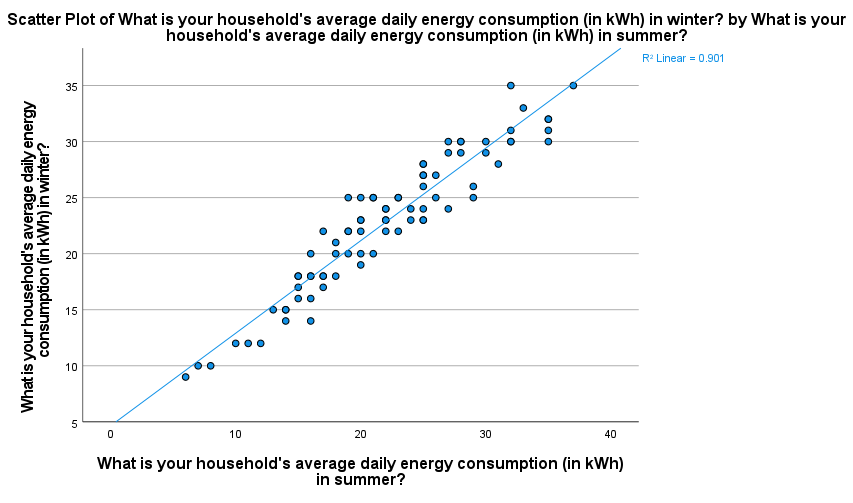This scatterplot shows that the relationship is approximately linear as the points lie close to the line of best fit. It also shows that the relationship is homoscedastic, as the points are a similar distance from the line of best fit all the way along (they don’t create a ‘funnel’ shape in either direction). Hence the fifth and sixth assumptions have been met.

1. If the assumptions are met, you can assess Pearson’s correlation coefficient in SPSS by choosing the following from the SPSS menu (either from the Data Editor or Output window):
• Analyze
• Correlate
• Bivariate
• select the variables (‘q6’ and ‘q7’) and move them into the Variables box
• click on OK

The output should look like this: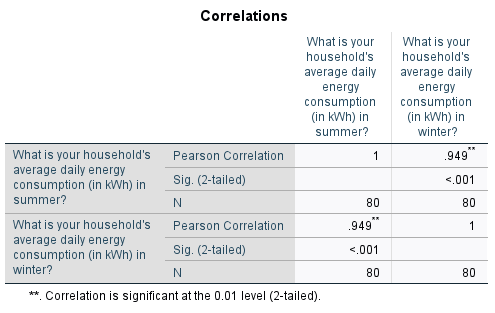1. This table shows that Pearson’s correlation coefficient is $$.949$$, indicating a strong positive linear correlation between summer and winter energy consumption (for more information on how to interpret this see the Descriptive statistics page of this module

To test whether this linear correlation is statistically significant requires the $$p$$ value (listed as ‘Sig. (2-tailed)’). Since $$p < .05$$ (in fact $$p < .001$$) we can reject the null hypothesis and conclude that there is a statistically significant linear correlation between summer energy consumption and winter energy consumption.

Pearson’s correlation coefficient and its square (the coefficient of variation) are also measures of effect size, which can be used to test for practical significance. The correlation coefficient of $$.949$$ indicates a large effect, and the coefficient of variation of $$90.06\%$$ indicates that $$90.06\%$$ of variation in winter energy consumption can be explained by variation in summer energy consumption.

For more information on how to interpret these results see the Introduction to statistics module.